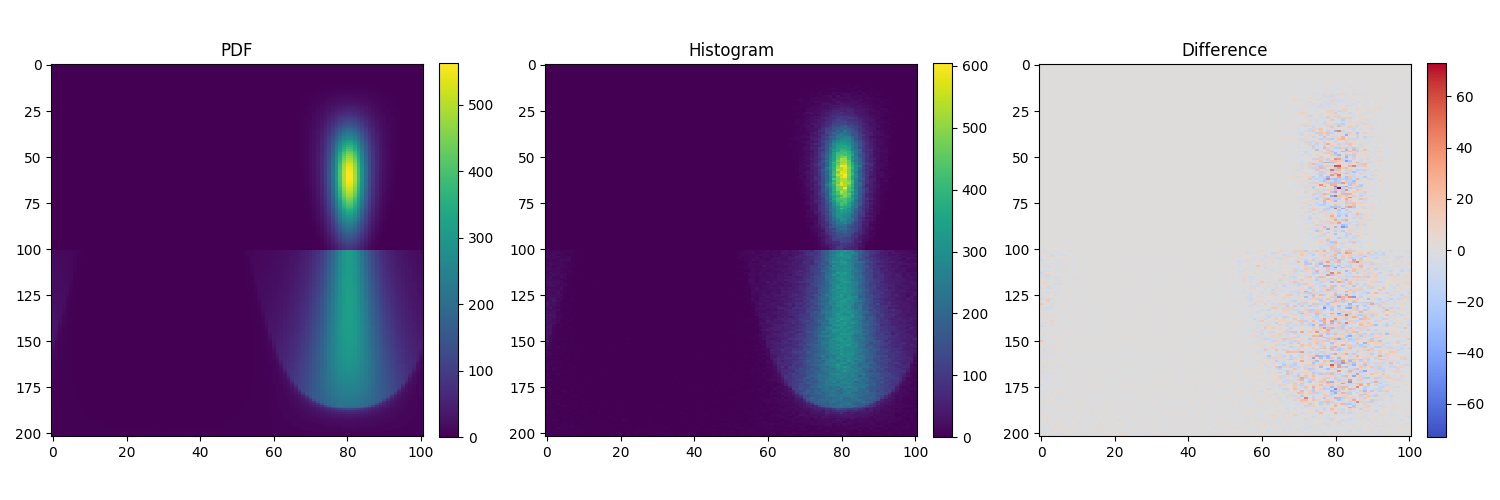# Testing#

To run the test suite, simply invoke pytest:

pytest

# or to run a single test file
pytest src/bsdfs/tests/test_diffuse.py


If you want to skip the execution of the slower tests, you can do so by running the test suite with the following flag.

pytest -m 'not slow'


The build system also exposes a pytest target that executes setpath and parallelizes the test execution.

ninja pytest


## Testing multiple variants#

The system provides a variety of Python fixture to automatically run unit tests on subsets of the available variants. Those should be passed a first argument to the test function like in the following example:

# This test will run on all available variants
def test_hello_world(variants_all):
print(f'Hello {mi.variant()}')
assert True


Here is the list of available fixtures:

• variants_all: execute on all variants

• variants_all_scalar: execute on all scalar_* variants

• variants_all_rgb: execute on all *_rgb variants

• variants_all_spectral: execute on all *_spectral variants

• variants_all_backends_once: execute the test for at least on variant per backend

• variants_all_ad_rgb: execute on all *_ad_rgb variants

• variants_all_ad_spectral: execute on all *_ad_spectral variants

• variants_any_scalar: execute on one scalar_* variant if available

• variants_any_llvm: execute on one llvm_* variant if available

• variants_any_cuda: execute on one cuda_* variant if available

• variants_vec_backends_once: execute on one cuda_* and one llvm_* variant if available

• variants_vec_backends_once_rgb: execute on one cuda_*_rgb and one llvm_*_rgb variant if available

• variants_vec_backends_once_spectral: execute on one cuda_*_spectral and one llvm_*_spectral variant if available

• variants_vec_rgb: execute on cuda_*_rgb and llvm_*_rgb variants

• variants_vec_spectral: execute on cuda_*_spectral and llvm_*_spectral variants

## Chi^2 tests#

The mitsuba.chi2 module implements the Pearson’s chi-square test for testing goodness of fit of a distribution to a known reference distribution.

The implementation specifically compares a Monte Carlo sampling strategy on a 2D (or lower dimensional) space against a reference distribution obtained by numerically integrating a probability density function over grid in the distribution’s parameter domain.

This is used extensively throughout the test suite to valid the implementation of BSDFs, Emitters, and other sampling code.

It is possible to test your own sampling code in the following way:

import mitsuba as mi
mi.set_variant('llvm_rgb')

# some sampling code
def my_sample(sample):
return mi.warp.square_to_cosine_hemisphere(sample)

# the corresponding probability density function
def my_pdf(p):
return mi.warp.square_to_cosine_hemisphere_pdf(p)

chi2 = mi.ChiSquareTest(
domain=mi.SphericalDomain(),
sample_func=my_sample,
pdf_func=my_pdf,
sample_dim=2
)

assert chi2.run()


In case of failure, the target density and histogram were written to chi2_data.py which can simply be run to plot the data:

python chi2_data.py


The mitsuba.chi2 module also provides a set of Adapter functions which can be used to wrap different plugins (e.g. BSDF, Emitter, …) in order to test them:

import mitsuba as mi
import drjit as dr

mi.set_variant('llvm_rgb')

xml = """<float name="alpha" value="0.5"/>
<boolean name="sample_visible" value="false"/>
<string name="distribution" value="ggx"/>
"""
wi = dr.normalize([0.2, -0.6, -0.5])
sample_func, pdf_func = mi.BSDFAdapter("roughdielectric", xml, wi=wi)

chi2 = mi.ChiSquareTest(
domain=mi.SphericalDomain(),
sample_func=sample_func,
pdf_func=pdf_func,
sample_dim=3
)

assert chi2.run()

# Forces the chi2 test to dump the plotting script (optional)
chi2._dump_tables()


Here is the figure generated by the chi2_data.py script from the example above:The plot on the left shows the density function generated by numerically integrating the analytical pdf() method of a roughdielectric BSDF with an incoming vector coming from inside. Most of the energy leaves the surface (upper half of the plot) while some energy gets reflected back inside the surface (lower half of the plot).

The middle plot shows the same density function but this time computed as a histogram of sampled directions resulting from the sample() method of the roughdielectric BSDF.

The right plot shows the difference between the two density functions. The sampling routine of the BSDF being stochastic, it is expected to see a mix of negative and positive values as the histogram is still noisy. The main role of the ChiSquareTest is to decide whether the observed deviation is within the range of random noise, or whether there are systematic biases that should lead to a test failure.

For more information, see mitsuba.chi2.ChiSquareTest.

## Rendering test suite and Z-test#

On top of test unit tests, the framework implements a mechanism that automatically renders a set of test scenes and applies the Z-test to compare the resulting images and some reference images.

Those tests are really useful to reveal bugs at the interaction between the individual components of the renderer.

The test scenes are rendered using all the different enabled variants of the renderer, ensuring for instance that the scalar_rgb renders match the cuda_rgb renders.

To only run the rendering test suite, use the following command:

pytest src/render/tests/test_renders.py


One can easily add a scene to the resources/data/tests/scenes/ folder to add it to the rendering test suite. Then, the missing reference images can be generated using the following command:

python src/render/tests/test_renders.py